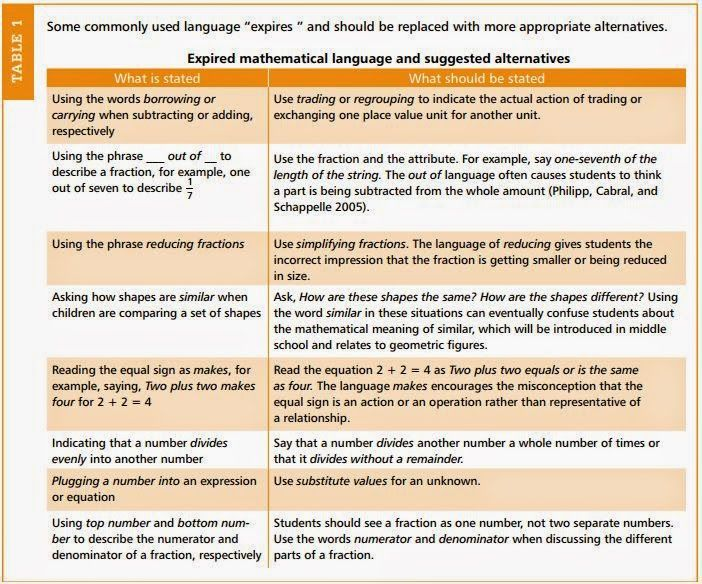Select Page

# “13 Rules That Expire”

Nov 6, 2014

Overgeneralizing commonly accepted strategies, using imprecise vocabulary, and relying on tips and tricks that do not promote conceptual mathematical understanding can lead to misunderstanding later in students’ math careers.http://www.nctm.org/

I found this article and it is worth sharing.  It outlines common rules and vocabulary that teachers share and elementary school students tend to overgeneralize—tips and tricks that do not promote conceptual understanding, rules that “expire” later in students’ mathematics careers, or vocabulary that is not precise.

Thirteen Rules that Expire:
1. When you multiply a number by ten, just add a zero to the end of the number.
2. Use key words to solve word problems.
3. You cannot take a bigger number from a smaller number.
4. Addition and multiplication make numbers bigger.
5. Subtraction and division make numbers smaller.
6. You always divide the larger number by the smaller number.
7. Two negatives make a positive.
8. Multiply everything inside the parentheses by the number outside the parentheses.
9. Improper fractions should always be written as a mixed number.
10. The number you say first in counting is always less than the number that comes next.This table shows some commonly used language that “expires” and more appropriate alternatives.  How many of these “rules” have you found yourself saying in the classroom?

So many of us are guilty of this at some point in our teaching career, but it’s not too late to stop old habits!